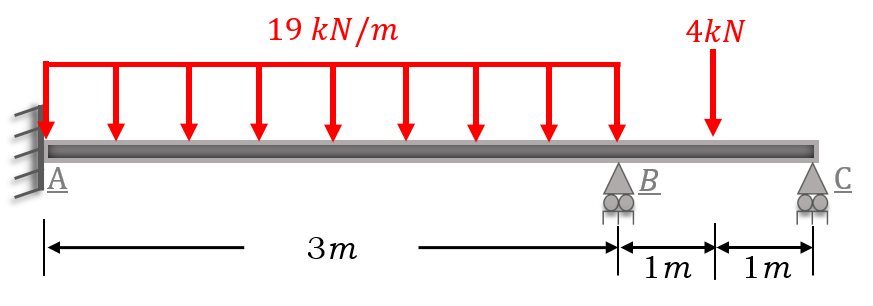# Beam Analysis by the Matrix Method of Rigidity | Excel ©HM.Beams V.1Example 1: Analyze the beam shown in the figure that is subjected to a distributed load and a point force, use the matrix method of stiffness.

EI = Constant

Solution:

a) Define the degrees of freedom and orientation of the element.

Note:

• The beam is not subjected to axial load, therefore two degrees of freedom are defined at each node (shear and moment).
• First we define the free degrees of freedom (green color).
• Second, we define the restricted degrees of freedom (red color).
• Finally, we define the orientation of the element.

b) Assemble the stiffness matrix of each element.

c) Assemble the stiffness matrix of the beam.

d) Partition of the sub matrix K₁₁.

Element 1

Element 2

f) Displacement vector calculation. |DD| = |K ₁|⁻¹ * |Cc|

g) Calculation of reactions. |CD| = |DD| * |K₂₁|

1.